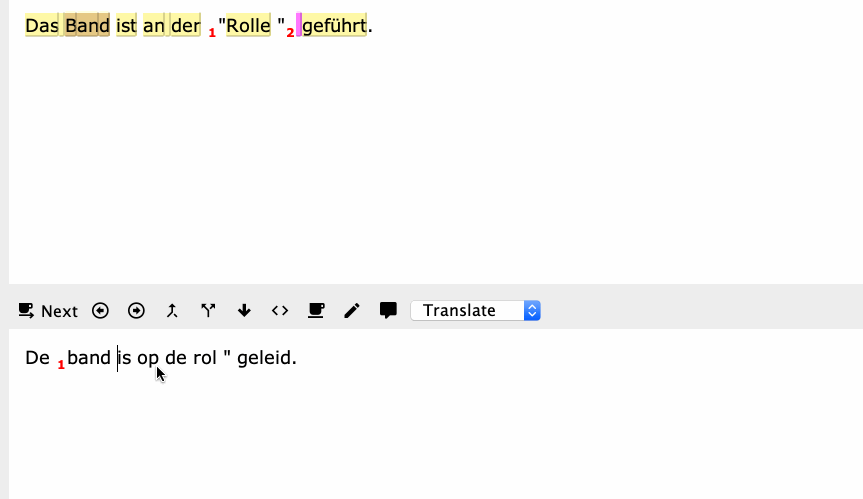Start a new topic

## KM macro: Surround quoted text with tagsTo automatically start auto-assembling in the next segment, you can add:Instead of moving forward and back, it's smarter and simpler to move back and forward again:Note that you have to deselect all skip settings for this to work.

Note that this macro will only work with PAIRED tags.

If one of the paired tags is missing, e.g. at segment start, won't work.

I've been trying to solve this with a negative look ahead for the ", but thus far, I haven't found a solution.

I’m afraid that the only solution for this will be to follow a much more complicated approach: first collect all tags from the source segment and save each and every tag in its own variable, or all in a collection. Then insert all saved tags at the correct positions in the target. Of course, this will only work for languages with similar syntax. The ideal situation would be if CTE would treat words as ‘magnetic’: all quotes and tags are glued to them—and to their translations.
Blij mee!!! Dat zijn heel veel JavaScript regels die een ontzettend vriendelijke, geduldige en hulpvaardige programmeur voor mij heeft geschreven. Ik heb ze vervolgens in een macro ingevoegd en nog wat simpele commando’s toegevoegd. Deze macro lost een probleem op dat ik als dom apenwerk beschouwde en waar ik dus een hekel aan had: dom rode tags invoegen, heel precies en saai werkje. En nu: één sneltoets en Keyboard Maestro doet het werk. Je snapt dat ik dit gisteravond nog even moest afronden

For those who want to have a look at the JavaScript:

(() => {

'use strict';

// Draft 003

// main :: IO ()

const main = () => {

const

kme = Application('Keyboard Maestro Engine'),

kmVar = k => kme.getvariable(k),

strEN = kmVar('strEN'),

strNL = kmVar('strNL');

const delims = '\"\'\“\”\‘\’{}()';

// delimSplit :: String -> [String]

const delimSplit = s =>

groupBy(on(eq)(c => delims.includes(c)))(s);

return zipWithLong(

en => nl => en.includes('<') ? (

`\${firstTag(en)}\${nl}\${lastTag(en)}`

) : delims.includes(nl) ? (

en

) : nl

)(

delimSplit(strEN)

)(

delimSplit(strNL)

).join('');

};

// firstTag :: String -> String

const firstTag = s =>

s.startsWith('<') ? (() => {

const i = [...s].findIndex(c => '>' === c);

return -1 !== i ? (

s.slice(0, 1 + i)

) : '';

})() : '';

// lastTag :: String -> String

const lastTag = s =>

s.endsWith('>') && s !== firstTag(s) ? (() => {

const

i = [...s].reverse().findIndex(

c => '<' === c

);

return -1 !== i ? (

s.slice(s.length - (1 + i))

) : '';

})() : ''

// --------------------- GENERIC ---------------------

// Tuple (,) :: a -> b -> (a, b)

const Tuple = a =>

b => ({

type: 'Tuple',

'0': a,

'1': b,

length: 2

});

// showLog :: a -> IO ()

const showLog = (...args) =>

console.log(

args

.map(JSON.stringify)

.join(' -> ')

);

// groupBy :: (a -> a -> Bool) -> [a] -> [[a]]

const groupBy = fEq =>

// Typical usage: groupBy(on(eq)(f), xs)

xs => (ys => 0 < ys.length ? (() => {

const

tpl = ys.slice(1).reduce(

(gw, x) => {

const

gps = gw,

wkg = gw;

return fEq(wkg)(x) ? (

Tuple(gps)(wkg.concat([x]))

) : Tuple(gps.concat([wkg]))([x]);

},

Tuple([])([ys])

),

v = tpl.concat([tpl]);

return 'string' !== typeof xs ? (

v

) : v.map(x => x.join(''));

})() : [])(list(xs));

// on :: (b -> b -> c) -> (a -> b) -> a -> a -> c

const on = f =>

// e.g. groupBy(on(eq)(length))

g => a => b => f(g(a))(g(b));

// eq (==) :: Eq a => a -> a -> Bool

const eq = a =>

// True when a and b are equivalent in the terms

// defined below for their shared data type.

b => {

const t = typeof a;

return t !== typeof b ? (

false

) : 'object' !== t ? (

'function' !== t ? (

a === b

) : a.toString() === b.toString()

) : (() => {

const kvs = Object.entries(a);

return kvs.length !== Object.keys(b).length ? (

false

) : kvs.every(([k, v]) => eq(v)(b[k]));

})();

};

// list :: StringOrArrayLike b => b -> [a]

const list = xs =>

// xs itself, if it is an Array,

// or an Array derived from xs.

Array.isArray(xs) ? (

xs

) : Array.from(xs || []);

// sj :: a -> String

function sj() {

// Abbreviation of showJSON for quick testing.

// Default indent size is two, which can be

// overriden by any integer supplied as the

// first argument of more than one.

const args = Array.from(arguments);

return JSON.stringify.apply(

null,

1 < args.length && !isNaN(args) ? [

args, null, args

] : [args, null, 2]

);

}

// zipWithLong :: (a -> a -> a) -> [a] -> [a] -> [a]

const zipWithLong = f => {

// A list with the length of the *longer* of

// xs and ys, defined by zipping with a

// custom function, rather than with the

// default tuple constructor.

// Any unpaired values, where list lengths differ,

// are simply appended.

const go = xs =>

ys => 0 < xs.length ? (

0 < ys.length ? (

[f(xs)(ys)].concat(

go(xs.slice(1))(ys.slice(1))

)

) : xs

) : ys

return go;

};

// MAIN --

return main();

})();

I’ll add a couple of lines to check whether the number of quotes in source and target are identical. Only then, the tags will be inserted.
I should also add a fix for isolated quotes that are surrounded by spaces. Like “ this” instead of “this”.